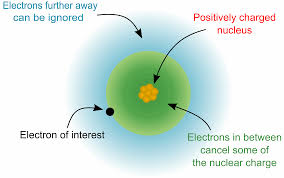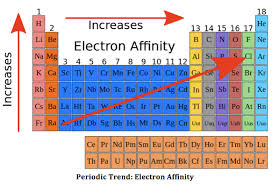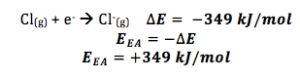# What is Meant By Electron Affinity Equation?

Electron affinity is the amount of energy changed (in kJ/mole) when an electron is introduced to a neutral atom in the gaseous state. The resultant reaction may be endothermic or exothermic and produces a negative ion. In other words, it is the capability of the positive nucleus of an atom to accept another negative electron within its orbital shell.The electronic affinity equation can be described in two stages. In the first stage, electron affinity is the amount of energy released to form 1 mole of negative ions.

X(g)+eX(g)

During the second stage, when the incoming electron is introduced to the negative ion, the amount of energy required is more than the energy released. Hence the equation is :

X(g)+eX2(g)

### What is Meant by Electron Affinity?Electron affinity is the ability of an atom to gain an incoming electron. It is the process of chemical reaction from which an atom loses or gains an electron. If the atoms gain an electron, an exothermic reaction takes place leading to the formation of a negative ion. But if an atom loses an electron, an endothermic reaction takes place forming positive ions.

### How To Write Electron Affinity EquationElectron affinity equation is the equation depicting the change in energy which occurs while converting 1 mole of an atom in its gaseous state into 1 mole of negative ion. When the additional electron is introduced for the first time to the neutral gaseous atom, it results in the release of heat or endothermic reaction.

Following is the first equation :

X(g)+eX(g)

During the second stage of electronic affinity, when the negative ion is introduced to another electron, the amount of energy required to incorporate another electron is greater than the energy released. Hence the atom loses its energy and in the process undergoes an exothermic reaction.

The second stage chemical reaction can be depicted through the following equation :

X(g)+eX2(g)

### First Electron Affinity Equation

When the first electron is introduced in the atomic orbital, there is a strong attraction between the positive nucleus and the negative electron. Hence the resultant chemical reaction produces heat and is an endothermic reaction.

This chemical reaction can be depicted through the following equation:

X(g)+eX(g)